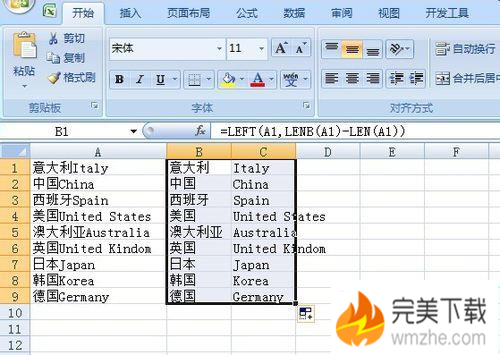# WPS中Excel实现中英文分列/分割详解LEN函数的含义是返回文本字符串的字符个数；

LENB函数的含义是返回文本中所包含的字符数，按照字节数计算，汉字、全角状态下输入的标点符号是两个字节长度；

A1的单位内容为：
zhangsan张三

=LEFT(A1,LENB(A1)-LEN(A1))

=RIGHT(A1,LEN(A1)-(LENB(A1)-LEN(A1)))